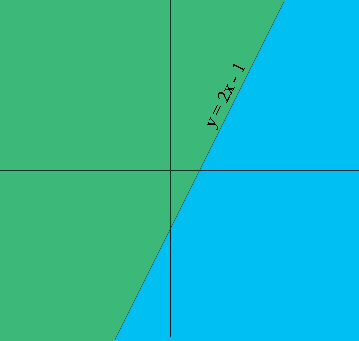SEARCH HOMEMath Central Quandaries & QueriesQuestion from Alice, a student: OK. I'm in algebra 1 and the linear inequality is y<2x-1 y>2 I don't completely know how to graph y>2 and I'm getting really frustrated Please help me in any way possible! Thank You! QuestionHi Alice,

A linear equation, such as $y = 2x - 1,$ partitions the plane into three parts, the line $y = 2x - 1$ and the two half planes $y < 2x - 1$ and $y > 2x - 1,$ one half plane on one side of the line and the other half plane on the other side of the line. In my diagram one half plane is shaded green and the other blue.To decide which half plane is described by the inequality $y < 2x - 1$ choose some point with simple coordinates that is not on the line. I am going to take $(0,0).$ Substitute the point $(0, 0)$ into the inequality to get $0 < -1.$ This is false so $(0, 0)$ is not in the half plane described by $y < 2x - 1.$ Thus the half plane described by $y < 2x - 1$ is the half plane shaded blue in my diagram.

For the inequality $y > 2,$ plot $y = 2$ and then choose some point not on this line, such as $(0, 0),$ from the diagram determine which alf plane it lies in and then check if it satisfies the inequality $y > 2.$

I hope this helps,
PennyMath Central is supported by the University of Regina and The Pacific Institute for the Mathematical Sciences.